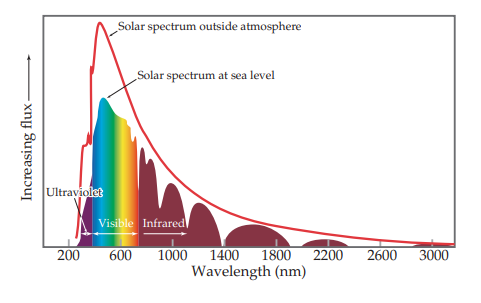×
Get Full Access to Chemistry: The Central Science - 14 Edition - Chapter 12 - Problem 12.75
Get Full Access to Chemistry: The Central Science - 14 Edition - Chapter 12 - Problem 12.75

×ISBN: 9780134414232 1274

## Solution for problem 12.75 Chapter 12

Chemistry: The Central Science | 14th Edition

• Textbook Solutions
• 2901 Step-by-step solutions solved by professors and subject experts
• Get 24/7 help from StudySoup virtual teaching assistantsChemistry: The Central Science | 14th Edition

4 5 1 423 Reviews
26
4
Problem 12.75

Silicon has a band gap of 1.1 eV at room temperature.

(a) What wavelength of light would a photon of this energy correspond to?

(b) Draw a vertical line at this wavelength in the figure shown, which shows the light output of the Sun as a function of wavelength. Does silicon absorb all, none, or a portion of the visible light that comes from the Sun?

(c) You can estimate the portion of the overall solar spectrum that silicon absorbs by considering the area under the curve. If you call the area under the entire curve “100%,” what approximate percentage of the area under the curve is absorbed by silicon?Step-by-Step Solution:
Step 1 of 3

CHM 031 Chemical Equilibria in Aqueous Systems Chapter 18 Notes Chapter 18 ​Electrochemistry: The Quest for Clean Energy 18.1 ​Running on Electrons: Redox Chemistry Revisited ❏ Electrochemistry​ - the branch of chemistry that examines the transformations between chemical and electrical energy ❏ Electrochemical cell​ - an apparatus that converts chemical energy into electrical work or electrical work into chemical energy ❏ Anode​ - an electrode at which an oxidation half-reaction (loss of electrons) takes place ❏ Cathode​ - an electrode at which a reduction half-reaction (gain of electrons) takes place 18.2 ​Voltaic and Electrolytic Cells ❏ Voltaic cell​ - an electrochemical cell in which chemical energy is transformed into electrical work by a spontaneous cell reaction ❏ Electrolysis​ - a process in which electrical energy is used to drive a nonspontaneous chemical reaction ❏ Electrolytic cell​ - a device in which an external source of electrical energy does work on a chemical system, turning reactant(s) into higher-energy product(s) ❏ Cell diagram​ - symbols that show how the components of an electrochemical cell are connected 18.3 ​Standard Potentials ❏ Standard reduction potential (​E​ )​ - the potential of a reduction half-reaction in which o​ all reactants and products are in their standard states at 25​ C ❏ Standard cell potential (​E​

Step 2 of 3

Step 3 of 3

##### ISBN: 9780134414232

The answer to “?Silicon has a band gap of 1.1 eV at room temperature. (a) What wavelength of light would a photon of this energy correspond to? (b) Draw a vertical line at this wavelength in the figure shown, which shows the light output of the Sun as a function of wavelength. Does silicon absorb all, none, or a portion of the visible light that comes from the Sun? (c) You can estimate the portion of the overall solar spectrum that silicon absorbs by considering the area under the curve. If you call the area under the entire curve “100%,” what approximate percentage of the area under the curve is absorbed by silicon?” is broken down into a number of easy to follow steps, and 110 words. Since the solution to 12.75 from 12 chapter was answered, more than 202 students have viewed the full step-by-step answer. Chemistry: The Central Science was written by and is associated to the ISBN: 9780134414232. This textbook survival guide was created for the textbook: Chemistry: The Central Science, edition: 14. The full step-by-step solution to problem: 12.75 from chapter: 12 was answered by , our top Chemistry solution expert on 10/03/18, 06:29PM. This full solution covers the following key subjects: . This expansive textbook survival guide covers 29 chapters, and 2820 solutions.

## Discover and learn what students are asking

Statistics: Informed Decisions Using Data : Estimating the Value of a Parameter
?State the properties of Student’s t-distribution.

Unlock Textbook Solution